# D105: in Heat Meter mode

### Pulse counter smart-MAIC D105 as Heat Meter

Based on the smart-MAIC D105 universal meter, you can create a Heat meter.
D105 in the Heat meter mode is designed for technical (non-commercial) accounting of the amount of heat produced or consumed in closed heat supply systems in apartments, cottages, enterprises and other premises.

#### Connecting D105 and sensors for heat meter operation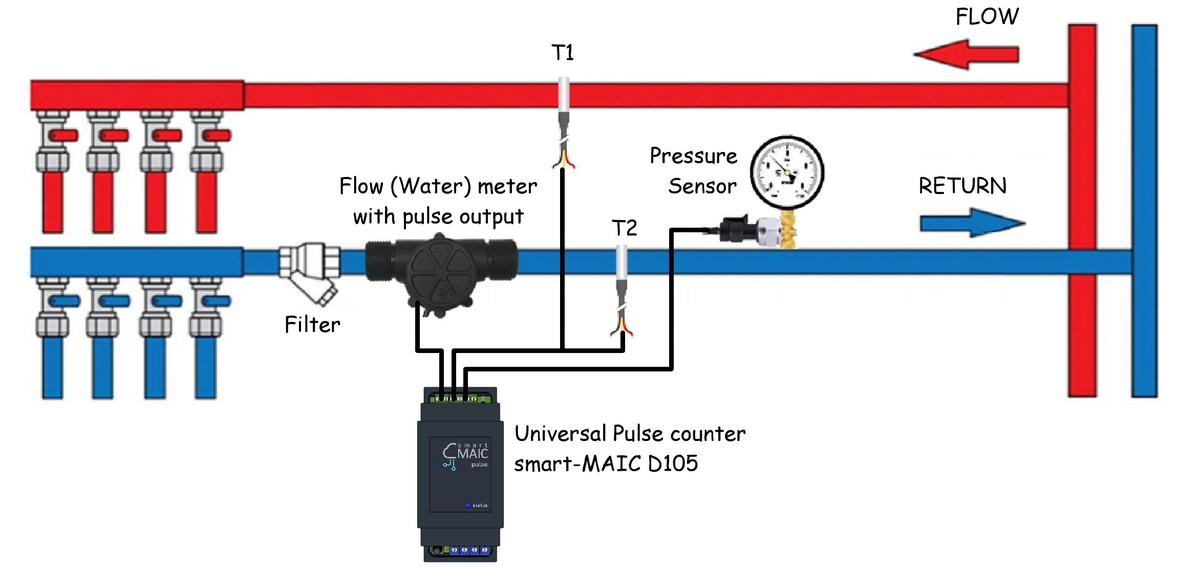##### To create a Heat meter you will need:
- D105 G2 and above, in the Extended version;
- Flow meter (hot water meter), with pulse output;
- Two temperature sensors DS18b20 at points T1 and T2;
- Optionally, a pressure sensor can be connected.

#### Activation of the Heat meter mode

1.The D105 universal meter must have an Extended license type.

You can check it in the device settings on the About page.
To get an Extended license, create a Support Ticket and indicate the device ID, an Extended license for D105 costs 10€.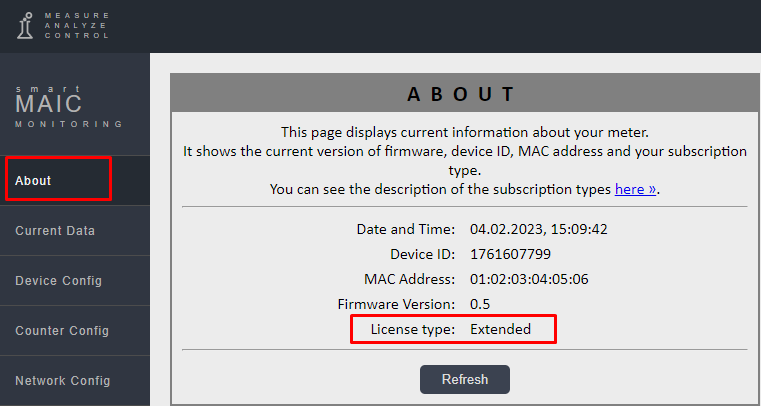2. In the "Counter Config" menu, set the operating mode to "Heat meter Ch2"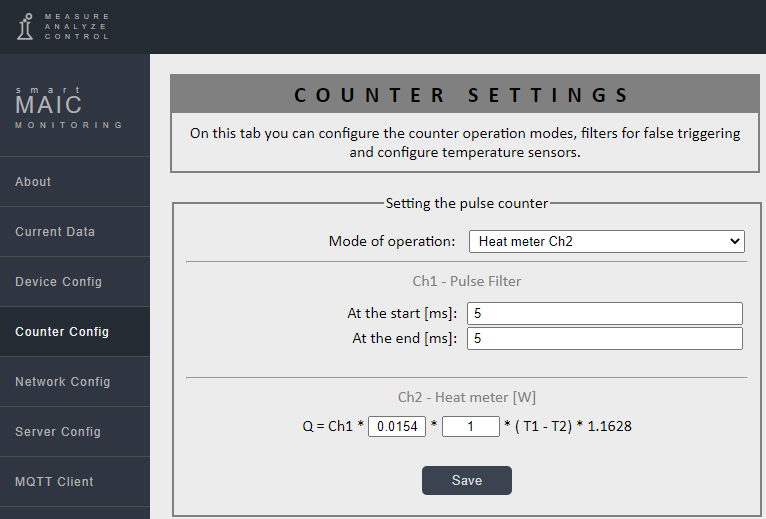#### Setting the Heat meter mode:

To determine the consumed / produced heat energy, a standard formula is used:

```[Kcal] Q =  m * k * (T1 – T2)
```

For reference: 1 Kcal = 1.1628 W; 1 Gcal = 1.1628 * 1000 * 1000 = 1162.8 kW; 1 W = 0.8598 Kcal;

The final formula for determining heat energy in Watts:
`[W] Q = m * k * (T1 – T2) * 1.1628`

Where:

m  - the mass of the spent coolant in kilograms.
k - thermal or correction factor. This may be the specific heat capacity of a substance or a correction factor for determining the mass of the coolant.
(T1 - T2) - temperature difference on the CH Flow and CH Return circuits of the heat carrier.

In the settings of the D105 device, the formula for determining heat energy is: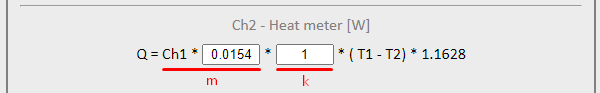To determine the mass of the coolant, you need a water meter with a pulse output, which must be connected to the D105 meter to the 1st pulse input [Ch1].
In the first field, you must specify the impulse price of the impulse per kilogram, i.e. how many kilograms of coolant are in 1 pulse. But on the meter, the pulse output usually counts liters, not kilograms. No worries, for water 1 liter = 1 kg.
If there are 10 liters per impulse, then the impulse price is 10.
If we have a meter where there are 65 impulses per 1 liter, then the impulse price is 1/65 = 0.0154

If the coolant is not water, you can use the correction factor [k] in the second field. For example, if the coolant is antifreeze and 1 liter of antifreeze = 1.09 kg, then the correction factor k = 1.09.
If there is a desire to make the formula even more accurate, then it is possible to determine the specific heat capacity of a substance, but it depends on its properties and temperature, and is found experimentally or from ready-made tables on the Internet.

Temperature sensors must be connected to D105 in strict accordance with the diagram above.
The CH Flow must be linked to the data [T1], and the CH Return to the data [T2].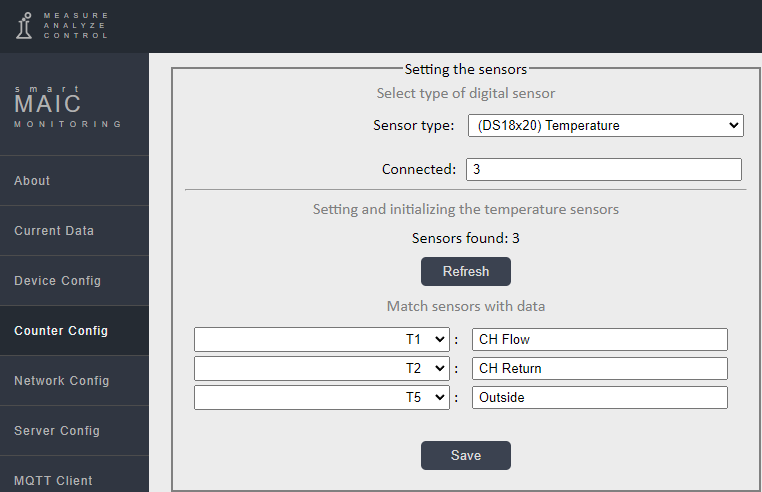Nothing can be connected to the 2nd pulse input of the D105 meter.

The produced/consumed heat energy in Watts will be stored in the data of the 2nd pulse input:
```[Ch2] - Watts of Heat per period minute/hour/day/month;
[TCh2] - Watts are cumulative, the value is constantly increasing, as on a simple meter.```

Done, use the data on the widgets on the Dashboard as usual to display indicators, tables and graphs in any available form.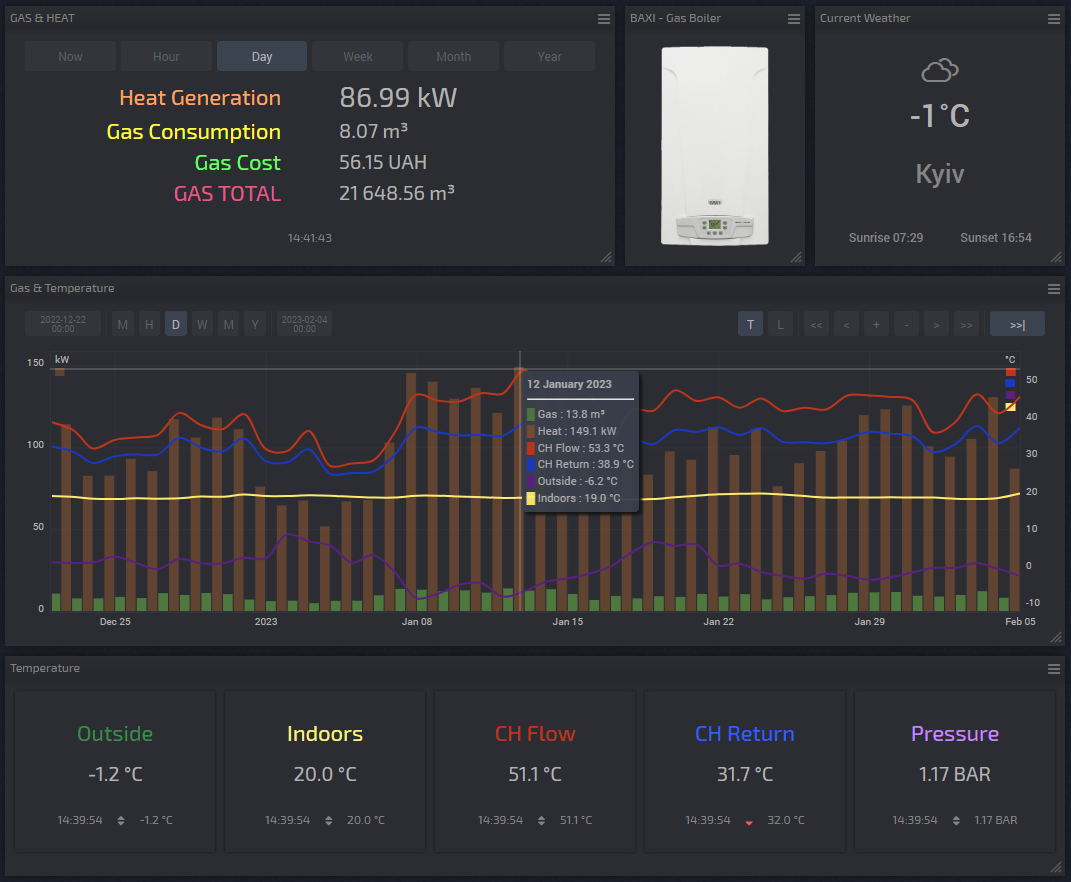Monitoring the operation of a Heat Pump (COP) Read »